Tamilnadu State Board New Syllabus Samacheer Kalvi 8th Maths Guide Pdf Chapter 6 Statistics Ex 6.3 Text Book Back Questions and Answers, Notes.

## Tamilnadu Samacheer Kalvi 8th Maths Solutions Chapter 6 Statistics Ex 6.3

Miscellaneous Practice Problems

Question 1.
Draw a pie chart for the given table.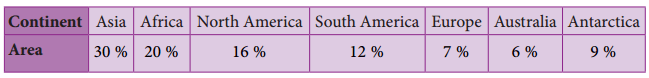Converting the area in percentage into components parts of 360°. we have.Continental Area.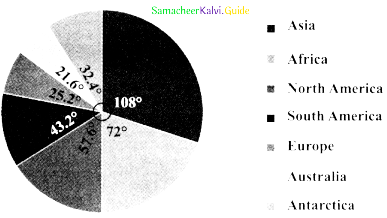Question 2.
The data on modes of transport used by the students to come to school are given below. Draw a pie chart for the data.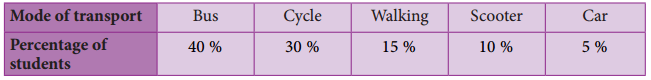Converting the percentage into components parts of 360°. we have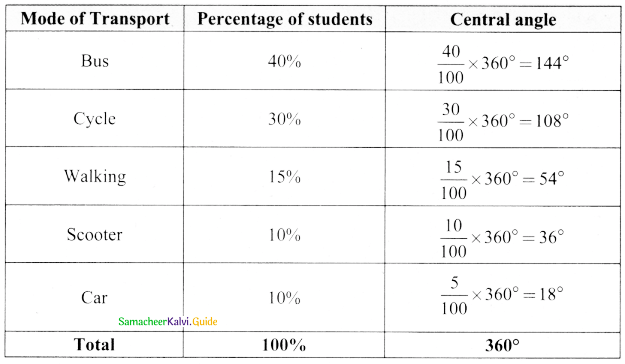Mode of Transport by students.Question 3.
Draw a histogram for the given frequency distribution.The given distribution is discontinuous.
Lower boundary = lower limit – $$\frac { 1 }{ 2 }$$ (gap between the adjacent class interval)
= 41 – $$\frac { 1 }{ 2 }$$(1) = 40.5
Upper boundary = Upper limit + $$\frac { 1 }{ 2 }$$ (gap between the adjacent class interval)
= 45 + $$\frac { 1 }{ 2 }$$(1) = 45.5
Now continuous frequency table is as belowQuestion 4.
Draw a histogram and the frequency polygon in the same diagram to represent the following data.The given distribution is discontinuous.
Lower boundary = lower limit – $$\frac { 1 }{ 2 }$$ (gap between the adjacent class interval)
= 50 – $$\frac { 1 }{ 2 }$$(1) = 49.5
Upper boundary = Upper limit + $$\frac { 1 }{ 2 }$$(gap between the adjacent class interval)
= 55 + $$\frac { 1 }{ 2 }$$(1) = 55.5
∴ The continuous frequency table is as below.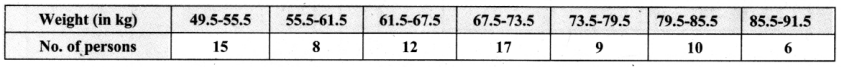Challenging problems

Question 5.
Form a continuous frequency distribution table and draw histogram from the following data.

 Age (in years) No of persons Under 5 1 Under 10 12 Under 15 19 Under 20 26 Under 25 27 Under 30 35 Under 35 38 Under 40 45 Under 45 48 Under 50 53

Converting into continuous distribution we have

 Class interval No. of persons 0 – 5 1 5 – 10 11 10 – 15 7 15 – 20 7 20 – 25 1 25 – 30 8 30 – 35 3 35 – 40 7 40 – 45 3 45 – 50 5 Total 53Question 6.
A rupee spent in a cloth manufacturing company is distributed as follows. Represent this in a pie chart.

 Particulars Paise Farmer 20 Spinner 35 Dyer 15 Weaver 15 Printer 05 Salary 10

1 Rupee = 100 paise.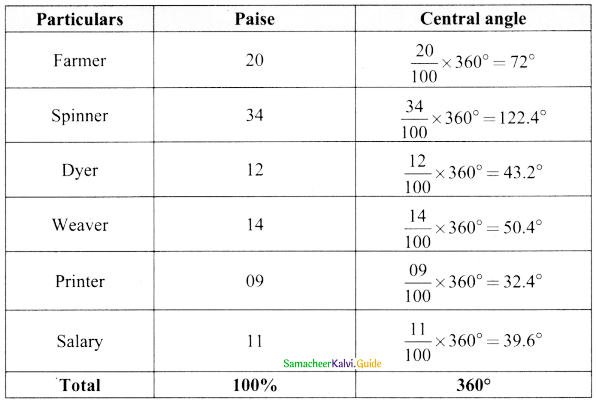Expenditure of a cloth manufacturing company.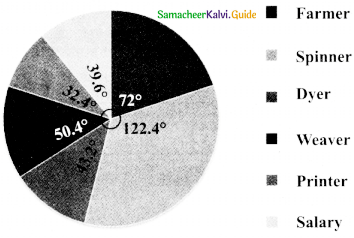Question 7.
Draw a histogram for the following data.Lower boundary lower limit – $$\frac { 1 }{ 2 }$$(gap between the adjacent class interval)
= 15 – $$\frac { 1 }{ 2 }$$(10) = 10
= 15 + $$\frac { 1 }{ 2 }$$(10) = 20